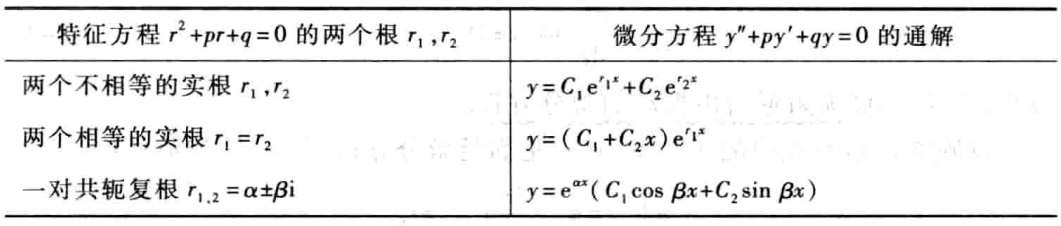# 高等数学(上册)第七章：微分方程

## 常系数齐次线性微分方程

• 1 当$p^{2}-4 q>0$时，$r_1,r_2$时两个不相等的实根

• 2 当$p^{2}-4 q=0$，$r_1,r_2$时两个相等的实根

• 3 当$p^{2}-4 q=0$，$r_1,r_2$是一对共轭复根

• 特征方程有两个不相等的实根

• 特征方程有两个相等的实根

• 特征方程有一对共轭复根：$r{1}=\alpha+\beta \mathrm{i}, r{2}=\alpha-\beta \mathrm{i}(\beta \neq 0)$。## 常系数非齐次线性微分方程

### $f(x)=e^{\lambda x} P_{m}(x)$型

• 如果$\lambda$不是(8-2)式的特征方程的根，即$\lambda^{2}+p \lambda+q \neq 0$，

• 如哦$\lambda$式特征方程的单根

• 如果$\lambda$使特征方程的重根

：求微分方程$y^{\prime \prime}-5 y^{\prime}+6 y=x \mathrm{e}^{2 x}$的通解。

## Final

If this post is helpful to you, why not reward me?
Author:
Reprint policy: All articles in this blog are used except for special statements CC BY 4.0 reprint polocy. If reproduced, please indicate source Gatsby !
Previous高等数学(下册)第九章：多元函数微分法及其应用

2020-03-06
Next高等数学(上册)第五章： 定积分

2020-02-08
TOC